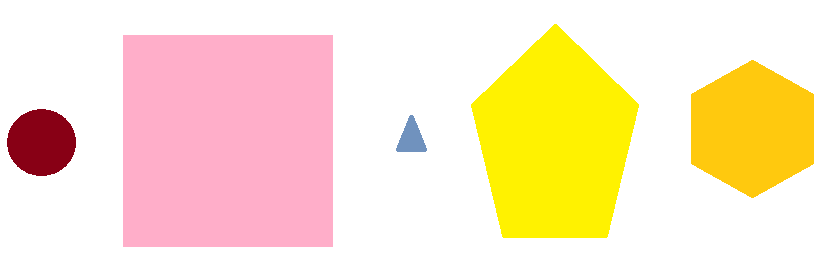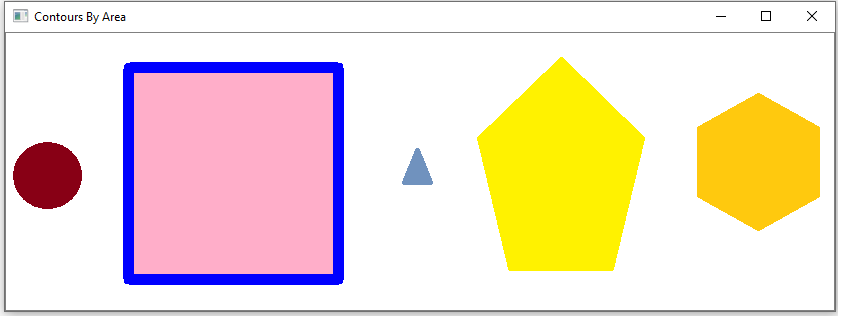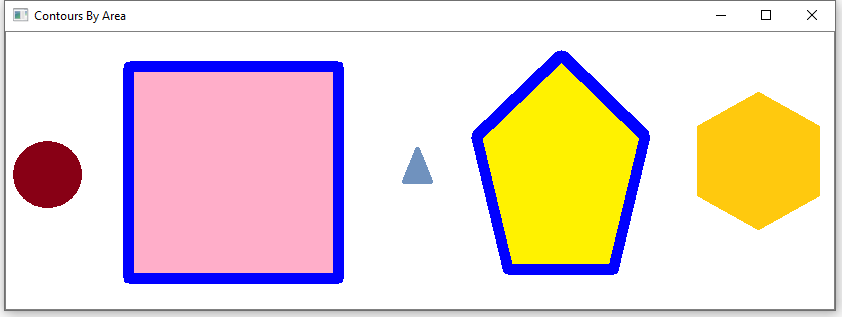﻿ How to Sort Objects in an Image By Size in Python using OpenCV ﻿# How to Sort Objects in an Image By Size in Python using OpenCVIn this article, we show how to sort objects in an image by size in Python using the OpenCV module.

OpenCV has functions in which it can locate and get the size of contours in an image.

We can then take these contours and organize them either from smallest to largest or from largest to smallest. Usually it's from largest to smallest, because we're most of all concerned with the larger parts of the images, while viewing smaller contours with less significance.

Contours represent various objects, or things, in an image. These may be a car, a person, a bicycle, etc.

In this example, we won't actually be sorting the items physically from, for example, left to right by size. Instead, we'll go through all the contours and sort them in a list. We can then do something like highlight the image one by one by adding a thick line around the contour.

In this example, we will be working with the following image shown below.As you can see in the above image, there are 5 shapes.

The largest is the square, followed by the pentagon, followed by the hexagon, followed by the circle, followed by the triangle.

In our code, we will create a list that has the contours sorted from largest to smallest.

We will draw outlines around each contour, one by one, from the sequence of the largest to the smallest.

This will create the following images shown below after each keypress.After a click, we get the following shown below.After another click, we get the following shown below.After another click, we get the following shown below.After another click, we get the following shown below.So you can see how we were able to sort objects from largest to smallest in Python using OpenCV.

Let's see now how this is done in code.

Let's now go over this code.

First, we import OpenCV using the line, import cv2

Next, we read in the image, which in this case is, Shapes.png

We create an original image because we modify our image throughout the code.

We then create a grayscale of the image and then the image with Canny edges.

We then get the contours of an image through the cv2.findContours() function. We are only concerned with the external contours of the image, as we are looking for the individual objects of our image and not the internal contours within an object.

We then display the image with the contours shown.

We then create a custom function, get_contour_areas(), which finds the areas of each of the contours within our image. This is done by using the cv2.contourArea() function.

We create an empty list, all_areas, which we will use to store each of the areas of each of the contours of the image.

We use a for loop to go through each of the contours, finding the areas of each, and then appending it to the all_areas list.

This function then returns the list of all_areas when executed.

We show the areas before sorting the list from largest to smallest.

We then sort the list from largest to smallest and store it in the sorted_contours variable. We sort it using the sorted() function with the key, cv2.contourArea. To sort it form largest to smallest, we set, reverse= True

We then show the sorted list from largest to smallest.

We then have a for loop that loops through the sorted_contours list, drawing outlines around each contour in the image.

When we run this code, this is the output we get through the various print commands.

So you can see how the contours are first unsorted and then they're sorted from largest to smallest.

If you want to sort the list from smallest to largest instead, you can either remove the line, reverse=True, or you can put, reverse= False

And this is how we can sort objects in an image by size in Python using OpenCV.

Related Resources